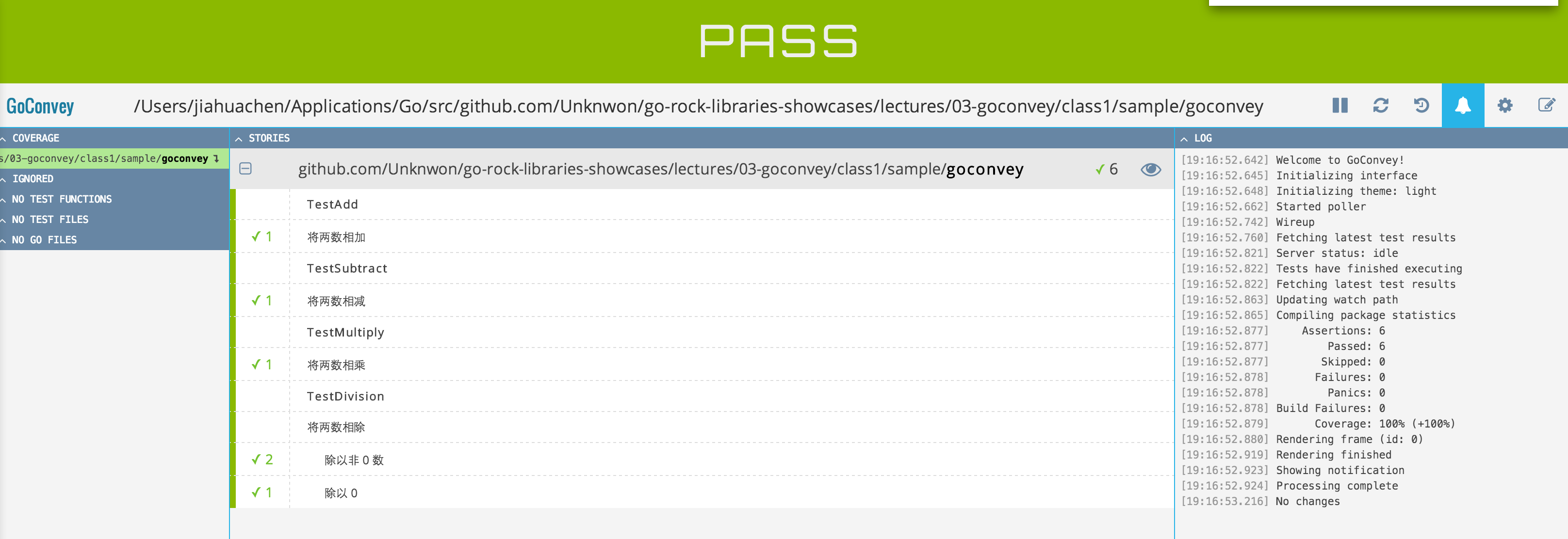# GoConvey - Go Testing Package: writing elegant tests

## Introduction

Go comes with built-in unit test feature, and there are lots of third-party helper libraries before GoConvey was born. Unfortunately, none of them can help you write elegant test cases like GoConvey does, simple syntax and comfortable interface make you fall in love with writing unit tests.

## Installation

``````go get github.com/smartystreets/goconvey
``````

### API Documentation

Please visit Go Walker.

## Basic Usages

### Write the code

Following code shows an example of basic four arithmetic(Add, subtract, multiply, divide):

``````package goconvey

import (
"errors"
)

func Add(a, b int) int {
return a + b
}

func Subtract(a, b int) int {
return a - b
}

func Multiply(a, b int) int {
return a * b
}

func Division(a, b int) (int, error) {
if b == 0 {
return 0, errors.New("can not divide by zero")
}
return a / b, nil
}
``````

In the code above, we implemented 4 functions, now we will use GoConvey to write test cases for them:

``````package goconvey

import (
"testing"

. "github.com/smartystreets/goconvey/convey"
)

func TestAdd(t *testing.T) {
Convey("Add two numbers", t, func() {
So(Add(1, 2), ShouldEqual, 3)
})
}

func TestSubtract(t *testing.T) {
Convey("Subtract one from another", t, func() {
So(Subtract(1, 2), ShouldEqual, -1)
})
}

func TestMultiply(t *testing.T) {
Convey("Multiply two numbers", t, func() {
So(Multiply(3, 2), ShouldEqual, 6)
})
}

func TestDivision(t *testing.T) {
Convey("Divide one from another", t, func() {

Convey("Divide by non-zero number", func() {
num, err := Division(10, 2)
So(err, ShouldBeNil)
So(num, ShouldEqual, 5)
})

Convey("Divide by zero", func() {
_, err := Division(10, 0)
So(err, ShouldNotBeNil)
})
})
}
``````

First of all, you should use the way that GoConvey team recommands to reduce redundant code: `. "github.com/smartystreets/goconvey/convey"`.

Every test function has to start with `Test`, such as `TestAdd`, and needs a `*testing.T` type argument.

To use GoConvey, every test case should use `Convey` function to start with. Its first argument is a `string` type argument; the second arguement usually is `*testing.T`, which is the varible `t` in this case; the third argument is a function with no argument and no return values(commonly use as closures).

You can have infinite nested `Convey` statements to present relationships between test cases. In the example, `TestDivision` function uses this way to shows the relations. Note that, only the out-most level need to pass varible `t`, and no need for other levels.

Finally, you can use `So` statement to test conditions. In this example, there are three different kinds of conditions: `ShouldBeNil`, `ShouldEqual` and `ShouldNotBeNil`, each represents value should be nil, values should equal, and value should not be nil. For more built-in conditions, please see Documentation.

### Run the tests

Now you can open the terminal and type `go test -v` to run the tests. Because GoConvey compatibles with Go built-in unit test, we can directly use Go commands to execute tests.

Following content comes from the output of test cases of the example(Mac):

``````=== RUN TestAdd

Add two numbers ✔

1 assertion thus far

--- PASS: TestAdd (0.00 seconds)
=== RUN TestSubtract

Subtract one from another ✔

2 assertions thus far

--- PASS: TestSubtract (0.00 seconds)
=== RUN TestMultiply

Multiply two numbers ✔

3 assertions thus far

--- PASS: TestMultiply (0.00 seconds)
=== RUN TestDivision

Divide one from another
Divide by non-zero number ✔✔
Divide by zero ✔

6 assertions thus far

--- PASS: TestDivision (0.00 seconds)
PASS
ok  	github.com/Unknwon/go-rock-libraries-showcases/lectures/03-goconvey/class1/sample/goconvey	0.009s
``````

As you can see, the output has very simple and understandable format, and test code are very elegant. But, is that all? Of course not! GoConvey has a very nice web interface to help automated testing as well.

### Web Interface

To enable web interface of GoConvey, you need to execute `goconvey`(install by `go get` to `\$GOPATH/bin`), then open the browser and visit http://localhost:8080:In the web interface, you can custom theme, see complete test results, browser notifications, etc.

Other features:

• Monitor code change and run tests
• Expressive DSL: http://localhost:8080/composer.html
• Coverage report: http://localhost:8080/reports/
• Stop monitoring a package

## Summary

At this point, you should have a sense of how to use GoConvey to write awesome test cases, and how it makes boring work to be interesting.

## Packages use GoConvey

To study in action, here is the list of packages that use GoConvey to write test cases: